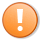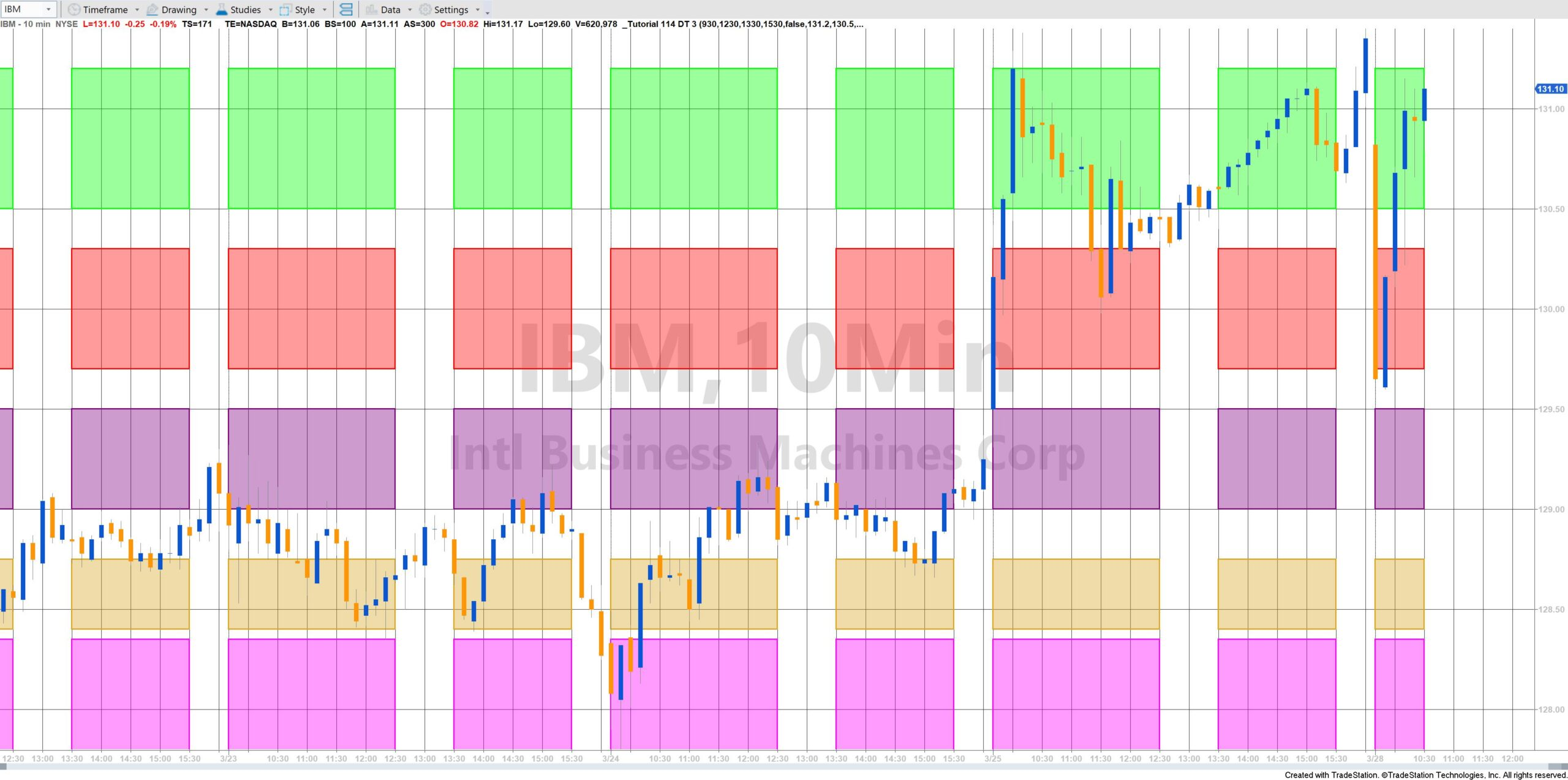# Tutorial 114 (DT) | Drawing boxes on a chart between time periods each day

Unlike the original _Tutorial 114 which draws boxes across the entire chart, _Tutorial 114 DT 3 draws boxes between two start and end times each day. If a chart does not have a bar with a specific time stamp corresponding to the start time the box will be drawn from the following bar. Similarly, if the chart does not have a bar corresponding to the end time, the box will be drawn to the following bar.

Drawing boxes might be useful if you wished to highlight trading activities and levels between certain times. Alternatively, although the times and price levels in this program are specified as user inputs, you could use the techniques built into this tutorial in a more sophisticated program that calculated levels.

The tutorial program uses the rectangle class with several of its properties and the create class. The tutorial demonstrates the creation of a method to create the rectangles, which is called from several places in the program. Tutorial 114 DT 3 creates five boxes at different price levels in each time zone.

A user input (ExtendRight) determines whether a box that is currently under development for real time bars is extended to the right or just extended to the current bar (until the end time is reached).

In situations where the start time is greater than the end time, the program assumes that the end time occurs the next day.

### Explanation of development

In the tutorial video and the program comments you will learn:

• How to write a method with several inputs
• How to use the rectangle class
• How to create a DT (DateTime) point
• How to extend the rectangles to the right
• Setting the end point of a rectangle
• How to vary the transparency of the rectangles
• How to draw historic and future rectangles on historic and real-time bars
• Specifying and using time to seconds accuracy
• Converting EasyLanguage legacy date and time into DateTime objects
• Dealing with start and end times that start in one day and end in the next
With this program it is easy to draw significant numbers of drawing objects. TradeStation limits the number of drawing objects that can be drawn on a chart.

### Inputs

double StartTime1( 1550 ), // Start time for box 1
double EndTime1( 1800 ), // End time for box 1
double StartTime2( 1900 ), // Start time for box 2
double EndTime2( 2200 ), // End time for box 2

bool ExtendRight( False ),

double Zone1_Hi( 1.133 ), // The price level of the upper edge of the rectangle for the first rectangle
double Zone1_Lo( 1.132 ), // The price level of the lower edge of the rectangle for the first rectangle
string Zone1_Color( “Blue” ), // The color of the first rectangle
int Zone1_Shading( 0 ), // The shading pattern of the first rectangle
int Zone1_Transparency( 100 ), // The alpha value of the first rectangle (between 0 and 255)

double Zone2_Hi( 1.131 ),
double Zone2_Lo( 1.13 ),
string Zone2_Color( “Red” ),
int Zone2_Transparency( 100 ),

double Zone3_Hi( 1.129 ),
double Zone3_Lo( 1.128 ),
string Zone3_Color( “Green” ),
int Zone3_Transparency( 100 ),

double Zone4_Hi( 1.127 ),
double Zone4_Lo( 1.126 ),
string Zone4_Color( “Goldenrod” ),
int Zone4_Transparency( 100 ),

double Zone5_Hi( 1.125 ),
double Zone5_Lo( 1 ),
string Zone5_Color( “Magenta” ),
int Zone5_Transparency( 100 );

### Code for Gold Pass members

Gold Pass members may copy and paste the following code into a new ShowMe study:This content is for members only.